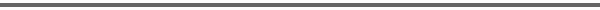Journal Home Page Cumulative Index List of all Volumes Complete Contentsof this Volume Previous Article Next Article Journal of Convex Analysis 11 (2004), No. 2, 285--301 Copyright Heldermann Verlag 2004Convergence of Convex Sets with Gradient Constraint Assis Azevedo Dept. of Mathematics, University of Minho, Braga, Portugal, assis@math.uminho.pt Lisa Santos Dept. of Mathematics, University of Minho, Braga, Portugal, lisa@math.uminho.pt[Abstract-pdf] \newcommand{\gd}{\nabla} \newcommand{\K}{\mathbb K} \newcommand{\N}{\mathbb N} \newcommand{\R}{\mathbb{R}} \newcommand{\wump}{{\bf W}^{1,p}_0(\Omega)} Given a bounded open subset of $\R^N$, we study the convergence of a sequence $(\K_n)_{n\in\N}$ of closed convex subsets of $\wump$ ($p\in]1,\infty[$) with gradient constraint, to a convex set $\K$, in the Mosco sense. A particular case of the problem studied is when $\K_n=\{v\in \wump: F_n(x,\gd v(x))\le g_n(x)\mbox{ for a.e.$x$in }\Omega\}$. Some examples of non-convergence are presented. We also present an improvement of a result of existence of a solution of a quasivariational inequality, as an application of this Mosco convergence result. FullText-pdf (475 KB) for subscribers only.